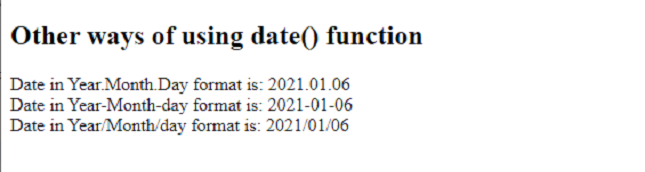GFG App
Open AppBrowser
Continue

# How to get the current Date and Time in PHP ?

The purpose of the article is to get the current Date and Time in PHP. It is performed by using a simple in-built PHP function date(). The Date is an inbuilt function used to format the timestamp. The computer stores date and time in UNIX timestamp. This time is measured as a number of seconds since Jan 1, 1970. As this is difficult for humans to read, PHP converts timestamps to format in such a way that it is readable and more understandable to humans.

Syntax:

`date('d-m-y h:i:s');`

Parameters :  The date() have different parameters. Each parameter represents some meaningful unit.

1. d: It represents the day of the month which has two digits with leading zeros (01 or 31)
2. m: It represents month in numbers with leading zeros (01 or 1)
3. y: It represents a year in two digits (08 or 14).
4. h: It represents the hour of the day in two digits with leading zeros (01 or 1)
5. I: It represents the minute in the current time zone.
6. s: It represents the number of seconds in the current timezone.

Example 1:

## PHP

 `  `

Output:

`Current date and time is :03-01-21 04:49:52`

Example 2: The following demonstrates other date() functions format which can be used by the developer as per the need.

## PHP

 ` ` ` ` ` ` `

Other ways of using ``date``() ``function``

` `"``; ` ` `  `echo` `"Date in Year-Month-day format is: "`  `    ``. ``date``(``"Y-m-d"``) . ``"
"``; ` ` `  `echo` `"Date in Year/Month/day format is: "`  `    ``. ``date``(``"Y/m/d"``) . ``"
"``; ` ` `  `?> ` ` ` ``

Output:My Personal Notes arrow_drop_up